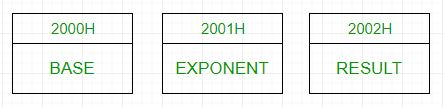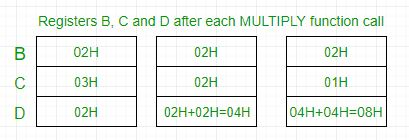Open in App
Not now

# 8085 program to find nth power of a number

• Last Updated : 07 Sep, 2018

Problem – Write an assembly language code for calculating the nth power of a number using 8085 microprocessor.

Example –

```Input : Base=>02H
Exponent=>03H
Output :08H
```In 8085 microprocessor, no direct instruction exists to multiply two numbers, so multiplication is done by repeated addition as 4*4 is equivalent to 4+4+4+4(ie 4 times).
Load 02H(base) to register B and 03H(exponent) to register C -> set D register to 02H -> Add 02H B(ie 2) times -> D register now contains 04H -> Add 04H B(ie 2) times -> D register now contains 08H -> Output is 08H.Algorithm –

1. Load the base into register B and exponent into register C.
2. To start multiplication set D to 01H.
4. Decrements C.
6. Take memory pointer to next location and store result.
7. Load E with contents of B and clear accumulator.
8. Repeatedly add contents of D to accumulator E times.
9. Store accumulator content to D.
10. Go to step 4.

Program –

2000H Base Data Byte for base
2001H Exponent Data Byte for exponent
2002H Result Result of factorial
2003H LXI H, 2000H Load data from memory
2006H MOV B, M Load base to B register
2007H INX H Increment memory
2008H MOV C, M Load exponent to C register
2009H MVI D, 01H Set D register to 1
200BH POWER_LOOP CALL MULTIPLY Subroutine call for multiplication
200EH DCR C Decrement C
200FH JNZ POWER_LOOP Call power_loop till C becomes 0
2012H INX H Increment memory
2013H MOV M, D Store result in memory
2014H HLT Halt
2100H MULTIPLY MOV E, B Transfer contents of B to E
2101H MVI A, 00H Clear accumulator to store result
2104H DCR E Decrement E
2108H MOV D, A Transfer contents of A to D
2109H RET Return from subroutine

Explanation –

1. Set register B with base and register C with exponent.
2. Set register D with base by calling MULTIPLY subroutine one time.
3. Decrement C and add D to itself B times by calling MULTIPLY subroutine.
4. Repeat the above step till C reaches 0 and then exit the program.
5. The result is obtained in D register which is stored in memory
My Personal Notes arrow_drop_up
Related Articles